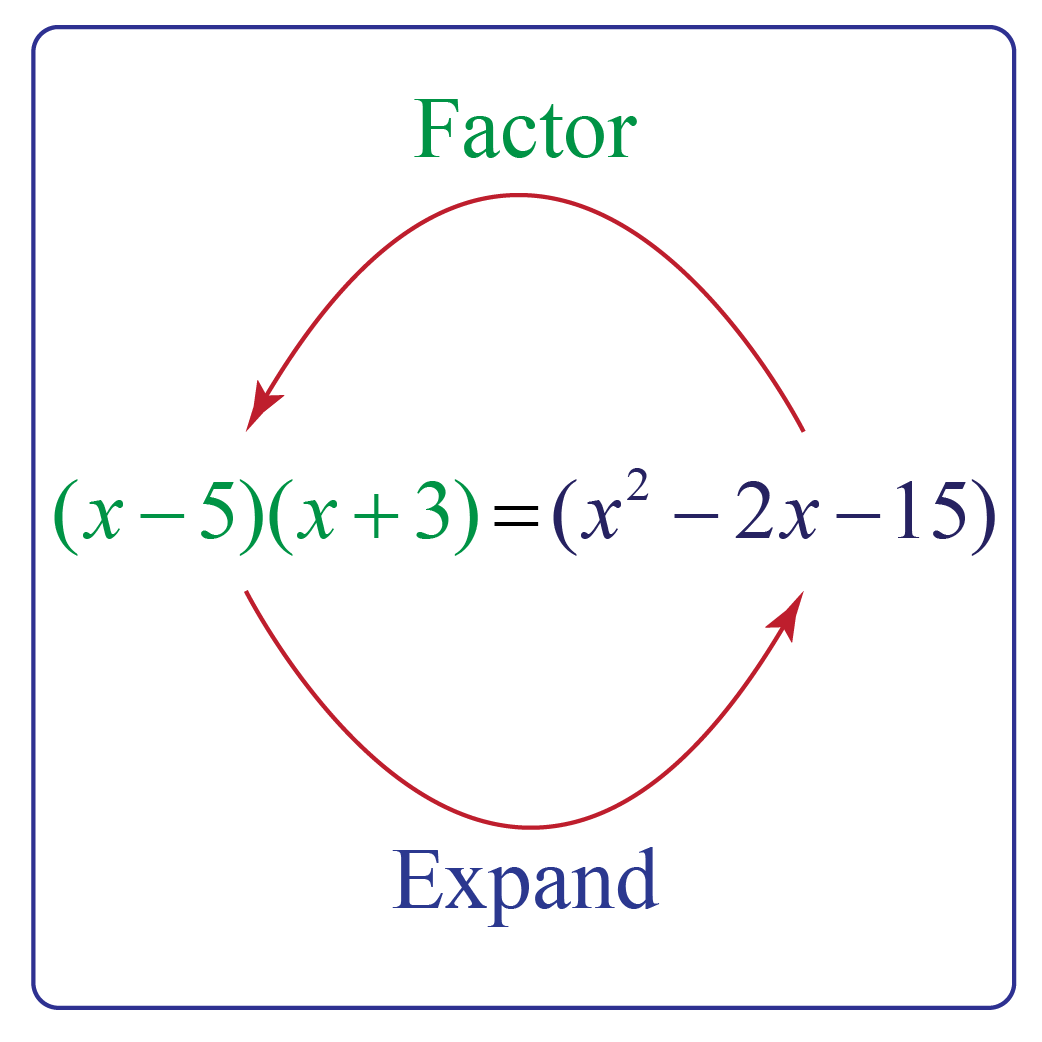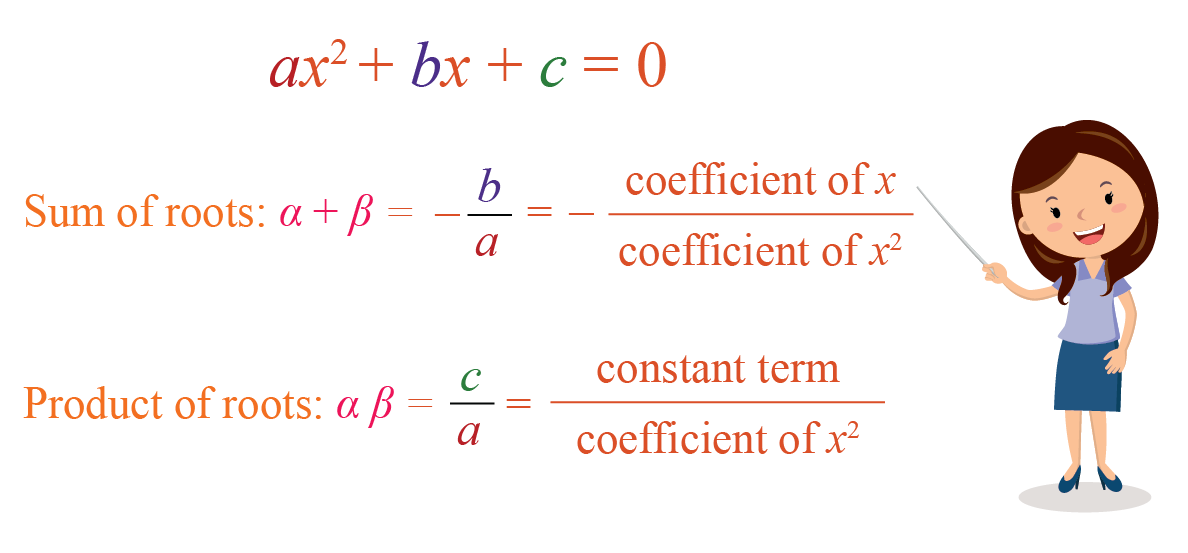We are aware of the fact that quadratic polynomials have 2 as their highest degree.

But do you know how to factorize a quadratic polynomial? Is it even possible?In this mini-lesson, we will explore the concept of factorization of quadratic polynomials. We will also learn what is a factor, common factor, quadratic polynomials, quadratic equation, how to find the value of k in polynomial, and examples of factorization of quadratic polynomials while discovering interesting facts about it.

## Lesson Plan

 1 What Is a Quadratic Polynomial? 2 Important Notes on the Factorization of Quadratic Polynomials 3 Solved Examples on the Factorization of Quadratic Polynomials 4 Challenging Questions on the Factorization of Quadratic Polynomials 5 Interactive Questions on Factorization of Quadratic Polynomials

## What Is A Quadratic Polynomial?

In a quadratic equation, a polynomial that has a degree of 2 is called a quadratic polynomial. It is also called a second-order polynomial.

The quadratic polynomial is in the form of $$f(x)=a_2x^2+a_1x+a_0$$.

A closed-form solution is known as the quadratic formula for the solutions of an arbitrary quadratic equation.

This indicates that the exponents of the variables in the quadratic polynomial must not be greater than 2

We get a quadratic equation after equating a quadratic polynomial to a constant.

An example of a quadratic polynomial is given in the image.## How To Factorize Quadratic Expressions?

Generally, factorization can be considered as the reverse of multiplying two expressions.

Let's take an example.

$$(x − 5)(x + 3) = x^2 − 2x − 15$$Here, we have multiplied two linear factors to obtain a quadratic expression by using the distributive law.

$$(x − 5)$$ and $$(x + 3)$$ are factors of $$x^2 − 2x −15$$

Let's check this to be sure.

\begin{align*}(x − 5)(x + 3) &= x(x + 3) +-5(x + 3)\\&= x^2 + 3x - 5x - 15\\=x^2 - 2x -15 \end{align*}

Thus, $$(x − 5)$$ and $$(x + 3)$$ are the factors of $$x^2 − 2x −15$$.

## Few Methods for Factorization of Quadratic Polynomials

### 1. Common Factor Method

In this method, we have to look at all the terms and determine the common terms.

If there is a common term in the equation, we will factor it out for the polynomial.

We use distributive law in reverse.
$x(a+b)=xa+xb$
We notice that each term has an 'x' in the equation and the common factor is taken out using the distributive law in reverse as follows,
$xa+xb=x(a+b)$

Example

What are the common factors of the terms in the equation $$8x^2 − 4x = 0$$?

Solution

Let's apply the distributive law in reverse.

4x is a common factor in the equation.

Thus, $$4x(2x-1)$$ are the factors of $$8x^2 − 4x = 0$$.

### 2. Sum of Difference Method

The sum and the difference of two terms is most likely used when the two factors match exactly, except one term involves addition and the other is a difference.

For example: (a+b)(a-b)

When we expand and multiply these terms, we get $$(a \times a+ab-ab-b \times b)$$

Like terms will be in the middle and will result in zero, thus leaving behind $$a^2$$ and $$-b^2$$.

Thus, the formula becomes $$(a+b)(a-b)=(a^2-b^2)$$.

Example

Find the solution of (5+x)(5-x) using the sum of the difference method.

Solution

Apply the sum of the difference method for solving the terms.
\begin{align*}(a+b)(a-b)&=(a^2-b^2)\\ (5+x)(5-x)&=(5^2-x^2)\\ &=25-x^2 \end{align*}

### 3. Product Sum Method

In the product sum method for factoring of polynomials, we use trinomials $$({ax^2}+bx+c)$$ where the value of a is 1.

This method is probably used the most for factoring polynomials.

If $$\alpha$$ and $$\beta$$ are the zeros of the quadratic polynomial \begin{align}&f\left( x \right):a{x^2} + bx + c \end{align}

The sum of the roots of the polynomial is: $- \dfrac{\text{coefficient of }x}{\text{coefficient of }x^2}$

In other words, it refers to:

 \begin{align} \alpha +\beta = -\frac{b}{a}\end{align}

The product of the roots of the polynomial is: $\frac{\text{constant term}}{\text{coefficient of }x^2}$

In other words, it refers to:

 \begin{align}\alpha \: \beta = \frac{c}{a}\end{align}Let’s take a look at an example.

Example

$p\left( x \right)= {x^2} - 13x + 42$

Determine the sum and product of the zeroes using the coefficients, and then verify your answer by factorization.

Solution

We have:

\begin{align}Sum &= - \frac{{{\rm{Coeff.}}\;{\rm{of}}\;x}}{{{\rm{Coeff.}}\;{\rm{of}}\;{x^2}}} = - \frac{{\left( { - 13} \right)}}{1} = 13\\Product &= \frac{{{\rm{Constant \: term}}}}{{{\rm{Coeff.}}\;{\rm{of}}\;{x^2}}} = \frac{{42}}{1} = 42\end{align}

Now, we factorize the polynomial by splitting the middle term:

\begin{align}p\left( x \right) &= {x^2} - 13x + 42\\& = {x^2} - 6x - 7x + 42\\& = x\left( {x - 6} \right) - 7\left( {x - 6} \right)\\& = \left( {x - 6} \right)\left( {x - 7} \right)\end{align}

Hence, the zeroes are 6 and 7.

 $$\therefore$$ Sum of the zeros is 13 and their product is 42

### 4. Factor by Grouping Method

Factor by grouping means that we have to group all the terms with common factors before factoring.

The following steps are used in the factor by grouping method.

• From the given expression, take out a factor from each group.
• Factorize each group of the expression.
• Now take out the factor common to the group formed.

Let’s take a look at an example.

Example

How can you factorize the algebraic expression $$a^2 - ac + ab - bc$$ by grouping method?

### Solution:

$$a^2 - ac + ab - bc$$

Take the common factor from the algebraic expression.
$= a(a - c) + b(a - c)\\= (a - c) (a + b)$

 $$\therefore$$ By factoring expressions, we get $$(a - c)(a + b)$$

5. Perfect Square Trinomials Method

The method of converting any trinomial into the perfect square is known as the perfect square trinomial method.

The following equations are the perfect square trinomial formulas:

 $a^{2}+2ab+b^{2}=(a+b)^{2}$
 $a^{2}-2ab+b^{2}=(a-b)^{2}$

Let’s take a look at an example.

Example

Is the given trinomial $$x^2 – 8x + 16$$ a perfect square?

Solution

On using the formula, we get

$${x^2} – 8x + 16 = {x^2} – 2(1)(4)x + {4^2}$$

$$= {(x – 4)^2}$$

The given binomial is a perfect square.

Thus, the given trinomial is a perfect square.

## Solved Examples

 Example 1

$q\left( x \right): 2{x^2} + 5x + 2$

Determine the sum and product of the zeroes using the coefficients, and then verify your answer by factorization.

Solution

We have:

\begin{align}&Sum = - \frac{{{\rm{coeff.}}\;{\rm{of}}\;x}}{{{\rm{coeff.}}\;{\rm{of}}\;{x^2}}} = - \frac{5}{2}\\&Product = \frac{{{\rm{constant \: term}}}}{{{\rm{coeff.}}\;{\rm{of}}\;{x^2}}} = \frac{2}{2} = 1\end{align}

Now, we factorize the polynomial by splitting the middle term:

$\begin{array}{l}q\left( x \right) = 2{x^2} + 5x + 2\\\;\;\;\;\;\,\,\, = 2{x^2} + 4x + x + 2\\\,\,\,\,\,\,\,\;\,\, = 2x\left( {x + 2} \right) + x + 2\\\,\,\,\,\,\,\,\;\,\, = \left( {2x + 1} \right)\left( {x + 2} \right)\end{array}$

The zeroes are:

$x = - \frac{1}{2},\;\;x = - 2$

Their sum and product is clearly the same as the values we obtained using the coefficients:

$\begin{array}{l}S = \left( { - \frac{1}{2}} \right) + \left( { - 2} \right) = - \frac{5}{2}\\P = \left( { - \frac{1}{2}} \right) \times \left( { - 2} \right) = 1\end{array}$

 $$\therefore$$ Sum = $$-\dfrac{5}{2}$$ and Product = 1
 Example 2

Samuel wants to find the common factors of the terms of the equation $$15x^2 − 5x = 0$$.

Can you help him find the answer?

Solution

5x is a common factor in the equation.

On taking it out, we get
$5x(3x-1)$

 $$\therefore$$ The factors of the expression are  $$(5x)$$ and $$(3x-1)$$
 Example 3

Consider the following quadratic polynomial: $$(5+k)(5-k)$$

Find the value of k using the sum of the difference method.

Solution

Apply the sum of the difference method for solving the terms.
\begin{align*} (a+b)(a-b)&=(a^2-b^2)\5+k)(5-k)&=(5^2-k^2)\\&=25-k^2\end{align*}  \( \therefore The solution of (5+k)(5-k) is  $$(5^2-k^2)$$ or $$25-k^2$$Challenging Questions
1. Determine a quadratic polynomial whose sum and product of its zeroes are 15 and 56 respectively.
2. The sum and product of the zeroes of a quadratic polynomial $$p$$ are 9 and 20 respectively.

Also, $$p\left( 6 \right) = 4$$.

Determine the polynomial $$p\left( x \right)$$.

3. Factorize the following polynomial by splitting the middle term:

$p\left( x \right):8{x^2} + 30x + 7$

## Interactive Questions

Here are a few activities for you to practice.

## Let's Summarize

The mini-lesson targeted the fascinating concept for factorization of quadratic polynomials. The math journey around the factorization of quadratic polynomials starts with what a student already knows, and goes on to creatively crafting a fresh concept in the young minds. Done in a way that not only it is relatable and easy to grasp, but also will stay with them forever. Here lies the magic with Cuemath.

At Cuemath, our team of math experts is dedicated to making learning fun for our favorite readers, the students!

Through an interactive and engaging learning-teaching-learning approach, the teachers explore all angles of a topic.

Be it problems, online classes, doubt sessions, or any other form of relation, it’s the logical thinking and smart learning approach that we, at Cuemath, believe in.

## 1. How do you solve quadratic equations by factoring?

We split the middle term into the factors of a number which we get by multiplying the first and the last term.

## 2. What are the different methods of factoring?

The different methods of factoring are:

• Factoring out the common factor
• The sum-product
• The grouping method
• The perfect square trinomial
• The difference of squares

## 3. What is the easiest way to factor an equation?

Splitting the middle term is the easiest way to factor an equation.

## 4. Is there any best way of factoring polynomials?

Applying the quadratic formula is the best way of factoring polynomials.

## 5. Where is factoring used in real life?

Dividing something into equal parts, managing time during traveling, price comparison, etc. is where factoring is used in real life.

## 6. What are the different types of factoring in math?

The different types of factoring in math are:

• The greatest common factor
• Grouping
• The difference in two squares
• Sum or difference in two cubes
• Trinomials
• General trinomials

## 7. What is quadratic factorization?

Quadratic factorization is the process of converting a quadratic polynomial into linear factors.

## 8. What do you mean by factorization of quadratic polynomials?

Factorization of quadratic polynomials generally means to find the value of the variable in the equation, also known as zeros of the polynomial.

## 9. How do you find the zeros of a quadratic polynomial?

We find the zeros of the polynomial by 4 different methods.

• Graphical method
• Completing the square method quadratic formula
• Splitting the middle term method
• Sum product method

## 10. How do you find the degree of a polynomial in the factored form?

The degree of a polynomial is the maximum power of the variable in the equation.

Polynomials
Polynomials
Grade 10 | Questions Set 1
Polynomials
Polynomials
Grade 9 | Questions Set 1
More Important Topics
Numbers
Algebra
Geometry
Measurement
Money
Data
Trigonometry
Calculus
More Important Topics
Numbers
Algebra
Geometry
Measurement
Money
Data
Trigonometry
Calculus